Related Articles
Heron’s Formula
• Last Updated : 19 Jan, 2021

Heron’s Formula is a very popular formula for finding the area of a triangle when the three sides are given. This formula was given by “Heron” in his book “Metrica”. We can apply this formula to all the types of triangles, be it right-angled, equilateral, or isosceles. The Heron’s Formula is,Where,

A = Area of Triangle ABC

a, b, c = Lengths of the sides of the triangle

s = semi-perimeter = (a + b + c)/2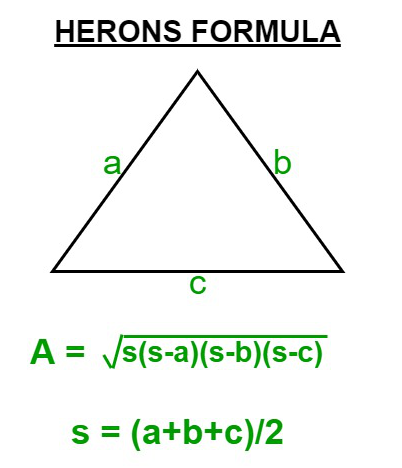Heron’s Formula

### Examples on Heron’s Formula

Example 1: Calculate the area of a triangle whose lengths of sides a,b and c are 14cm,13cm, and 15 cm respectively?

Solution:

Given:- a = 14cm

b = 13cm

c = 15cm

Firstly, we will determine semi-perimeter(s)

s = (a + b + c)/2

s = (14 + 13 + 15)/2

s = 21cmExample 2: Find the area of the triangle if the length of two sides is 11cm and 13cm and the perimeter is 32cm?

Solution:

Let a, b and c be the three sides of the triangle.

a = 11cm

b= 13 cm

c =?

Perimeter = 32cm

As we know, Perimeter equals to the sum of the length of three sides of a triangle.

Perimeter = (a + b + c)

32 = 11 + 13 + c

c = 32 – 24

c= 8cm

Now as we already know the value of perimeter,

s = perimeter/2

s = 32/2

s =16cm

a = 11cm, b = 13cm, c = 8cm, s = 16cm## Surface Area

The area occupied by the surface of the solid object can be called a surface area. Generally, the Area is of two types:

1. Total Surface Area
2. Lateral Surface Area

We will discuss both of them in detail in the latter part of the article.

## CUBE

A solid three-dimensional object whose length, breadth, and height all are equal is called a cube. A cube consists of  6 faces, 12 edges & 8 vertices.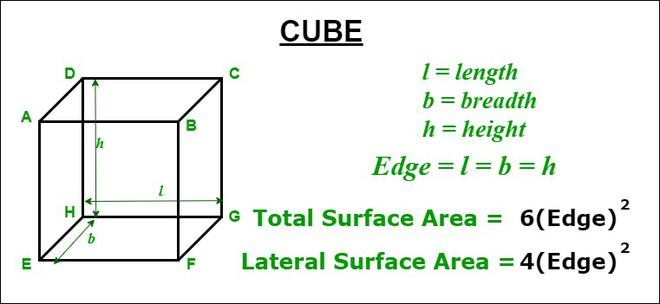Cube

### Formula 1: Total Surface Area of cube = Sum of its six faces

Deriving the formula for the surface area of the cube:

Consider a cube of length l cm, breadth b cm and height h cm.

Area of face ABCD = Area of face EFGH = (l * b)cm2

Area of face AEHD = Area of face BFGC = (b * h)cm2

Area of face ABFE = Area of face DHGC = (l * h)cm2

As we know, Total Surface Area of Cube = Sum of the all the areas of all it’s six faces

Total Surface Area = 2(l * b) + 2(b * h) + 2(l * h)cm2

= 2(l * b + b * h + h * l)cm2

= 2(lb + bh + hl)cm2

As we know, length, breadth and height of a cube are always equal.

= 2(l * l + l * l + l * l)cm2

2 ∗ 3l2 cm2

Total Surface Area = 6(Edge)2

### Example

Find the surface area of a cube whose edge is 8cm?

Solution:

As we know, Total surface area of cube6(Edge)2

here, Edge = 8

Total Surface Area 6 ∗ (8)2

Total Surface Area of the cube is 384 cm2

### Formula 2: Lateral Surface Area of Cube = Sum of the areas of four faces(Leaving the bottom and top faces)

Lateral Surface Area Of Cube = Area of face AEHD + Area of face BFGC + Area of face ABFE + Area of face DHGC

Lateral Surface Area = 2(b * h) + 2(l * h) cm2

As we know, all sides of the cube are equal

Lateral Surface Area of cube = 2(l * l + l * l) cm2

Lateral Surface Area Of cube = 4l2 = 4(Edge)2

### Example

Find the cost of painting a cubical box of side 6cm which is open from the top at the rate of 5cm/sq?

Solution:

Side = 6cm

Lateral Surface Area of box 4(Edge)2

Lateral Surface Area = 4∗ (6)2

The lateral surface area of box = 144 cm2

Cost of painting = 5 cm2

Total cost = 144 * 5 = 720 Rupees

## CUBOID

A solid three-dimensional object having length, breadth, and height with six rectangular faces placed at the right angle. Like, cube a cuboid also consists of 6 faces, 12 edges & 8 vertices.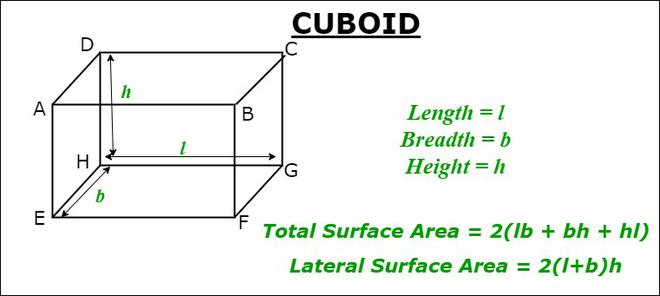Cuboid

### Formula 1: Total Surface Area of cuboid = Sum of its six faces

Deriving the formula for the surface area of the cuboid:

Consider a cuboid of length l cm, breadth b cm and height h cm.

Area of face ABCD = Area of face EFGH = (l * bArea of face AEHD = Area of face BFGC = (b * hArea of face ABFE = Area of face DHGC = (l * hAs we know, Total Surface Area of Cuboid = Sum of the all the areas of all it’s six faces

Total Surface Area = 2(l * b) + 2(b * h) + 2(l * h)Total Surface Area of Cuboid= 2(l * b + b * h + h * l)Total Surface Area of Cuboid = 2(length * breadth + breadth * height + height * length)### Formula 2: Lateral Surface Area of Cuboid = Sum of the areas of four faces(Leaving the bottom and top faces

Lateral Surface Area of Cuboid = Area of face AEHD + Area of face BFGC + Area of face ABFE + Area of face DHGC

Lateral Surface Area of Cuboid  = 2(b * h) + 2(l * h)Lateral Surface Area of Cuboid  = 2(l + b) * hLateral Surface Area of Cuboid  = 2(Length + Breadth) * Height### Example

Calculate the Total surface area and Lateral Surface area of a chalk box of length, breadth, and height 16cm, 8cm, and 6cm respectively?

Solution:

As we know that chalk box is cuboidal in shape.

Given, length = 16cm, breadth = 8cm, height = 6cm

Total Surface Area = 2(length * breadth + breadth * height + height * length)

Total Surface Area = 2(16 * 8 + 8 * 6 +16 * 6)Total Surface Area = 2(128 + 48 + 96)Total Surface Area = 544Lateral Surface Area = 2(Length + Breadth) * Height

Lateral Surface Area = 2(16 + 8) * 6Lateral Surface Area = 288## RIGHT CIRCULAR CYLINDER

A solid shape generated by the revolution of a rectangle about one of its sides is called a right circular cylinder. Example: Straw, Rubber Pipes. A solid bounded by two coaxial cylinders of the same height and different radius is called a hollow cylinder.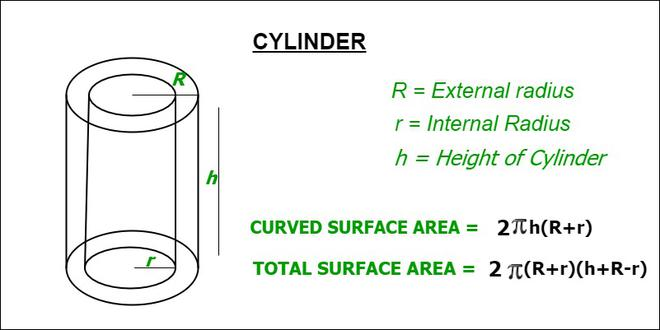Cylinder

Let R and r be the external and internal radius of a hollow cylinder and h be their height.

### Formula 1: Total Surface Area = External Surface Area + Internal Surface Area + Area of top + Area of base

Deriving the formula of the surface area of a Right Circular Cylinder:

External Surface AreaInternal Surface AreaArea of topArea of baseTotal Surface Area =Total Surface Area =Total Surface Area### Formula 2: Curved(Lateral) Surface Area = External Surface Area + Internal Surface Area

Curved Surface Area =Curved Surface Area### Example

Find the Total Surface Area and Curved Surface Area of a hollow right circular cylinder of height 14 cm and Internal radius = 2cm and External radius = 3cm?

Solution:

Given, Height = 14cm , r = 2cm , r = 3cm

Total Surface Area =Total Surface Area =Total Surface AreaAs we know the value of= 3.14(approx)

Total Surface Area = 471Curved Surface Area =Curved Surface Area = 2*3.14*14*5

Curved Surface Area = 439.82## RIGHT CIRCULAR CONE

A Three-Dimensional solid object having a flat base and an apex. Example: Birthday Caps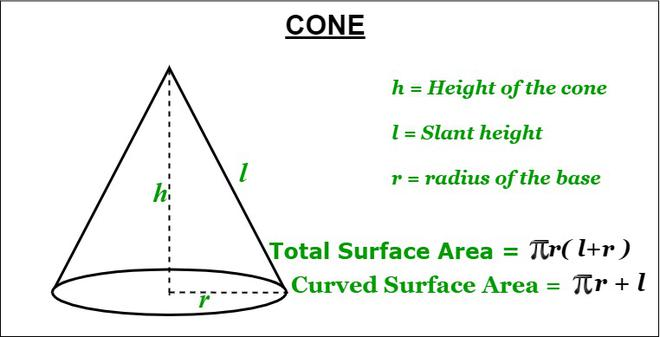Cone

Height = The length of the line joining a vertex to the centre of the base.

Slant height = The length of the line joining a vertex to any point of the circular edge.

Radius = Radius of the base.

Deriving Formula for the Surface area of Right Circular Cone :

Let there be a cone of radius r, height h and slant height l.

Therefore, Length of Circular edgeArea of the plane =### Formula 1: Total Surface Area Of Cone = Area of the Sector + Area of the Base

Area of the Sector = 1/2 * (arc length) * (radius)

Area of the SectorArea of the BaseTotal Surface Area =Total Surface Area### Formula 2: Curved(Lateral) Surface Area Of Cone = Area Of the Sector

Curved Surface Area### Example

Find the Total Surface Area and Curved Surface Area of a Cone of slant height 9cm and diameter 14cm?

Solution:

Given,  slant height(l) = 9cm,  radius(r) = diameter /2 = 7cm

Curved Surface Area =Curved Surface Area = 3.14 * 7 * 9Curved Surface Area = 197.9Total Surface Area = Curved Surface Area + Area of the Base

Total Surface Area = 197.9 +Total Surface Area = 197.9 + 153.9 = 351.83## SPHERE

A Three-Dimensional solid object having all its points equidistant from a fixed point and is round in shape. Example: Ball.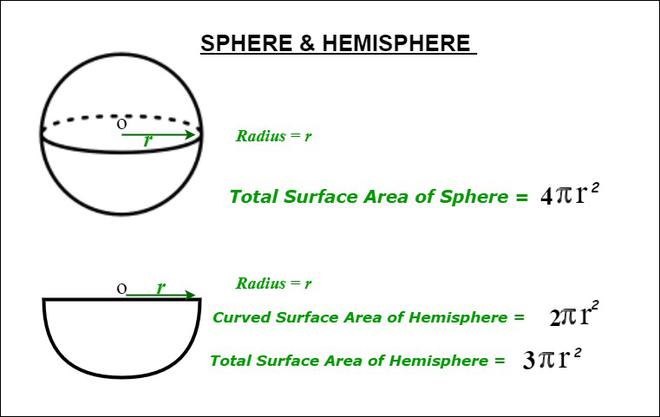Sphere & Hemisphere

Note: The sphere has only total surface area.

Let, there be a sphere of radius r.

Surface Area of Sphere =Hemisphere

A plane through the center of a sphere divides the sphere into two equals parts. Each of them is called a hemisphere.

Curved Surface Area Of Hemisphere =Total Surface Area of Hemisphere = Curved Surface Area + Area of Base

Total Surface Area of hemisphere =Total Surface Area of Hemisphere### Example

Find the area of a sphere of radius 6cm also find the Total Surface Area and Curved Surface Area if the sphere divided into two equal halves?

Solution:

Given,  radius(r) = 6cm

Area of Sphere =Area of sphere = 4 * 3.14 * 6 * 6Area of sphere = 452.38We know if we divide a sphere into two equal parts, we eventually get two hemispheres of the same area.

Curved Area of the HemisphereCurved Surface Area = 2 * 3.14 * 6 * 6 = 226.19Total Surface Area of Hemisphere =Total Surface Area of Hemisphere = 3 * 3.14 * 6 * 6 = 339.2Attention reader! Don’t stop learning now. Get hold of all the important DSA concepts with the DSA Self Paced Course at a student-friendly price and become industry ready.

My Personal Notes arrow_drop_up